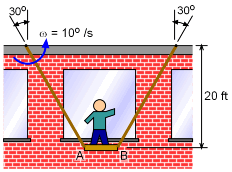Ch 5. Rigid Body General Motion Multimedia Engineering Dynamics Fixed Axis Rotation Plane Motion Velocities Zero Velocity Point Plane Motion Accelerations Multiple Gears Rot. Coord. Velocities Rot. Coord. Acceleration
 Chapter - Particle - 1. General Motion 2. Force & Accel. 3. Energy 4. Momentum - Rigid Body - 5. General Motion 6. Force & Accel. 7. Energy 8. Momentum 9. 3-D Motion 10. Vibrations Appendix Basic Math Units Basic Equations Sections Search eBooks Dynamics Fluids Math Mechanics Statics Thermodynamics Author(s): Kurt Gramoll ©Kurt GramollDYNAMICS - CASE STUDY IntroductionProblem Diagram Jim Knowitall just started a new job washing windows. He decides to spread the two ropes further apart at the top so the platform will be more stable (or so he thinks). What is known: A wind gust causes the platform to swing. The ropes rotate at a constant angular velocity of 10°/s counterclockwise. The current vertical distance is 20 feet. The ropes are 30° off the vertical. The platform is initially horizontal and is 6 feet wide. Questions What is the angular velocity of the platform when the ropes are spread at 30° to the vertical? Is it safe? Approach One approach is to use the relative velocity equation, as was done in the previous section. A second, and sometimes easier approach is to use the concept of "Instantaneous Center of Zero Velocity" (IC). To use the IC method, the velocities of any two points on the body are needed.

Practice Homework and Test problems now available in the 'Eng Dynamics' mobile app
Includes over 400 problems with complete detailed solutions.
Available now at the Google Play Store and Apple App Store.